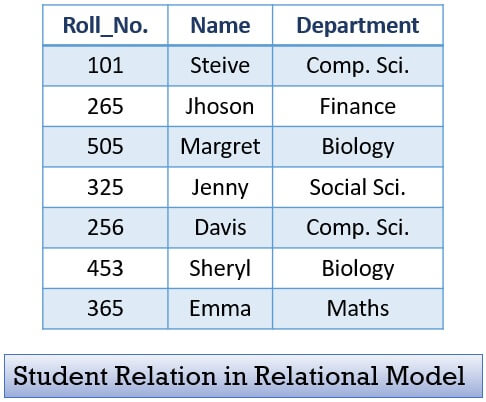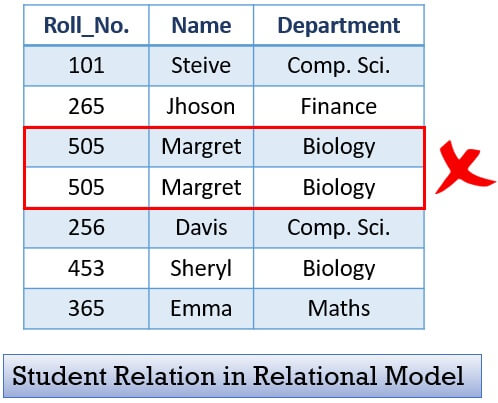# Relational Data Model

The relational data model provides conceptual tools to design the database schema of the relational database. The relational model describes the data, relationship between that data, data sematic and constraints on the data in the relational database.

The relational model expresses the data and relationship among the data in the form of tables. There is a lot more to discuss about, the relational model. In this section, we will discuss the relational model, its diagram, its characteristics, its constraints, advantages & disadvantages in detail.

## Content: Relational Model in DBMS

### What is Relational Model?

Relational Data Model was first prosed by Ted Codd of IBM in the 1970s. But, its commercial implementations were observed in the 1980s. The relational data model is employed for storing and processing the data in the database.

The building blocks of the relational model has been developed on the concept of mathematical relation. And its theoretical concepts are based on set theory and first-order predicate logic. The relational model is also referred to as the record-based model as it frames the database in fixed-format records of various types.

A relational model is popular for its simplicity and possibility of hiding the low-level implementation details from database developer and database users.

Relational data model expresses the database as a set of relations (table of values). Each relation has columns and rows which are formally called attributes and tuples respectively. Each tuple in relation is a real-world entity or relationship. The name of the relation and the name of attributes contribute to interpreting the sense of each tuple. Like it expresses what kind of entities does a relation have?

Let us count points to understand the relational model in short:

• The database is a set of related relations (table of values).
• Each relation has a name which indicates what type of tuples the relation has. For example, a relation named ‘Student’ indicates that it has student entities in it.
• Each relation has a set of attributes (column names) which represents, what type of information, the entities or tuples have? For example, Student relation has a set of attributes Roll_No., Name, Department. It indicates that the Student relation has student entities/tuples that have information about their roll_no., name and department.
• A tuple (row) in a relation, is a real-world entity, it has a set of values for corresponding attributes.
• Each data value in a row or tuple is called field.

### Relational Model Diagram

The figure below indicates a relation in a relational model.It is a Student relation and it is having entries of 5 students (tuples) in it. The figure below will help you identify the relation, attributes, tuples and field in a relational model.### Characteristics of Relational Database Model

As we know we have several relations in a database. Now, each relation must be uniquely identified. If it is not so, then it would create a lot of confusion. Here, we will discuss some characteristics that when followed will automatically make a relation distinct in a database.

1. Each relation in a database must have a distinct or unique name which would separate it from the other relations in a database.

2. A relation must not have two attributes with the same name. Each attribute must have a distinct name.

3. Duplicate tuples must not be present in a relation.4. Each tuple must have exactly one data value for an attribute. For example, below in the first table, you can see that for Roll_No. 265 we have enrolled two students Jhoson and Charles, this would not work. We must have only one student for one Roll_No.5. Tuples in a relation do not have to follow a significant order as the relation is not order-sensitive.

6. Similarly, the attributes of a relation also do not have to follow certain ordering, it’s up to the developer to decide the ordering of attributes.

### Relational Model Constraints

Relational model constraints are restrictions specified to the data values in the relational database. Initially, we will describe the constraints on the database, they are categorized as follows:

• Inherent Model-Based Constraints: The constraints that are implicit in a data model are inherent model-based constraints. For example, a relation in a database must not have duplicate tuples, there is no constraint in the ordering of the tuples and attributes.
• Schema-Based Constraints: The constraints that are specified while defining the schema of a database using DDL are schema-based constraints. They are further categorized as domain constraints, key constraints, entity integrity constraints, referential integrity constraints and constraints on Null Value.
• Application-based Constraints: The constraints that cannot be applied while defining the database schema are expressed in application programs. For example, the salary of an employee cannot be more than his supervisor.

Now let us explore the Schema-based constraints in detail:

• Domain Constraints:
Each attribute in a tuple is declared to be of a particular domain (for example, integer, character, Boolean, String, etc.) which specifies a constraint on the values that an attribute can take.
• Key Constraint and Constraint on Null Values:
In relation, a key can either be a single attribute or a subgroup of attributes that can recognize a particular tuple in a relation. Now, the key constraint specifies that a key (attribute/subset of attribute) must not have the same set of values for the tuples in a relation.
The constraint on NULL values defines whether an attribute is allowed to carry Null value or not. For example, in a student tuple, its name attribute must be NOT NULL.
• Entity Integrity Constraint:
Entity integrity constraint specifies that a primary key of a tuple can never be NULL. As primary key used to identify individual tuple in a relation.
• Referential Integrity Constraint:
The referential integrity constraint holds if the foreign key of relation R1 that refers to the relation R2 satisfies following two conditions:

1. The set of attributes that form foreign key of relation R1 should have the same domain as the primary key of the referenced relation R2.
2. In the current state, the set of values of the foreign key in tuple t1 of relation R1 must match a primary key value in referenced relation R2 or it could be NULL.

1. It is the simplest and easy to use, data model.
2. It hides the physical storage details from the database developers and database users.
3. It is scalable as you can keep adding records and attributes to records in a database.